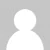# Sort Comparison Functions That Return Boolean Value is Deprecated in PHP 8.0

PHP provides functions that allows to sort an array using a user-defined comparison function. The comparison function must return an integer greater than zero if first element is larger than second, less than zero if first element is smaller than second, or zero if both elements are equal:

• > 0 if a > b
• < 0 if a < b
• 0 if a = b

Sorting functions that accept a user-defined comparison function is summarised in the following table:

Since PHP 8.0, if comparison function returns a boolean value (`true` or `false`), PHP will emit a deprecation warning.

``````<?php

\$array = [5, 1, 9, 3, 5];
usort(\$array, function (int \$a, int \$b): bool {
return \$a > \$b;
});

print_r(\$array);``````

Example will output:

``````Deprecated: usort(): Returning bool from comparison function is deprecated, return an integer less than, equal to, or greater than zero in main.php on line 6
Array
(
 => 1
 => 3
 => 5
 => 5
 => 9
)``````

Example can be rewritten using spaceship operator (`<=>`). It returns -1, 0 or 1 when first element is respectively less than, equal to, or greater than second element.

``````<?php

\$array = [5, 1, 9, 3, 5];
usort(\$array, function (int \$a, int \$b): int {
return \$a <=> \$b;
});

print_r(\$array);``````

1.2.